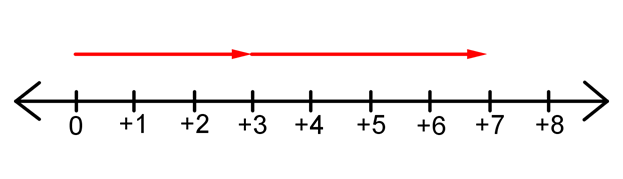#### All You Need in One Place

Everything you need for better marks in primary, GCSE, and A-level classes.#### Learn with Confidence

We’ve mastered the UK’s national curriculum so you can study with confidence.#### Instant and Unlimited Help

0/1
0/21
##### Examples
###### Lessons
1. What addition statement does each of the following diagrams represent? Then, find the sum.

1.2.3.2. Use a number line to add.
1. (+2) + (+4) =
2. (+10) + (-7) =
3. (-8) + (-3) =
4. (-6) + (+5) =
3. The temperature in city A was +28°C. The temperature then dropped by 13°C at midnight. What was the final temperature?
1. A diver was diving 2 m under the water. He descended 8 m and then ascended 4 m. What was his final depth under the water?
1. Adding 1-digit Numbers Vertically – Same Signs
1. $(+2)+(+4)$
2. $(-5)+(-3)$
3. $(+8)+(+6)$
4. $(-9)+(-4)$
2. Adding 1-digit Numbers Vertically – Opposite Signs
1. $(-7)+(+1)$
2. $(-7)+(+9)$
3. $(+5)+(-2)$
4. $(+5)+(-8)$
1. $(+36)+(+28)$
2. $(-39)+(-54)$
3. $(+12)+(-43)$
4. $(-22)+(+63)$
0%
##### Practice
###### Topic Notes
In this section, we will use our knowledge from previous section to build our understanding of integer addition. We will be asked to write addition statements using arrows on number lines. A thermometer is just like a number line. In this section, the blue arrows on the number lines will move to the left, or down the number line, and represent negative integers. A negative integer on a thermometer would represent a cooler temperature. In this section, the red arrows will move to the right, or up the number line, and represent positive integers. A positive integer on a thermometer would represent a warmer temperature.

You see numbers in your everyday life all the time. Numbers can be either positive or negative. When you see a number written without a "+" or "-" sign, that is, without a sign, it usually means that the number is positive. For example, if you saw the number 6 exactly the way we wrote it here, it means positive 6.

Adding positive numbers involve just simple addition, and subtracting them is just simple subtraction.

## How to add negative numbers

When we talk about negative numbers, things get a bit more difficult. A negative number has a "-" sign in front of it. For example, -6 would mean negative 6.

What happens when we try to add two negative numbers? Two negatives actually make a positive! When you see two minus signs next to each other, you'll know to add it instead. Why does it work like this? Let's take an example dealing with your bank account.

If you've got $10 in the bank, but the bank accidentally removed$5, it'd look like: $10 -$5 = $5. You'd be left with$5 in your account. Since it was the bank's mistake and they want to take away a mistake (the -$5), it'll look like$5 - (-$5) =$5 + $5 =$10. They're remove the negative (the mistake in this case), which in the end, makes it a positive, so you'll end up with \$10 in your bank again.

An example of two negatives in real life can be seen through double negatives when we're speaking. If we told you to not cross the road, it would mean just that. But if we told you to not not cross the road, it means you should cross the road. In a similar sense, you can use this to help you when adding negative numbers.

A good rule of thumb is that when you see two like signs, add the numbers together (i.e. two "-" signs, or two "+" signs). If you see unlike signs (i.e. one "-" and one "+"), it becomes a negative and you'll have to subtract it.

## Adding and subtracting integers examples

Let's try some number line examples to see how adding and subtracting positive and negative integers work.

Question 1:

What addition statement does each of the following diagrams represent? Then, find the sum.

Solution:

In this question, the first arrow = +3.

The second arrow = +4.

This is an addition statement and the final sum of the numbers gives us 7.

Question 2:

Use a number line to add

(+2) + (+4)=

Solution:

The first term we have is a +2. This means that the first arrow we'd draw will represent +2.

The second arrow we draw represents +4.

Then we'll get the final answer of landing on 6 on the number line.

Question 3:

Use a number line to add

(-8) + (-3)=

Solution:

Our first arrow represents -8

The second arrow we will draw represents -3

In the end, we'll get a total of -11.

If you need to check your calculations on positive and negative integer calculation, try out this online calculator.

Looking for more topics to review? Learn what the order of operations are with PEMDAS, and how to deal with subtraction and addition of decimal numbers. There are models to help you add and subtract fractions as well such as adding fractions with like denominators. You can also try your hand at divisibility rules that can help you find factors of whole numbers.

In this lesson, we will learn:

• Adding 1-digit Numbers Vertically – Same Signs
• Adding 1-digit Numbers Vertically – Opposite Signs